Example Questions

Example Question #1 : Solve Radical Equations

Solve for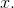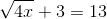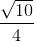Explanation:

In order to solve this equation, we need to isolate the radical. In order to do so, we subtract 3 from both sides which leaves us with: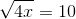To get rid of the radical, we square both sides: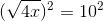the radical is then canceled out leaving us with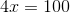We solve forby dividing by 4: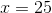Example Question #1 : Radical Functions

Solve for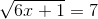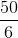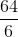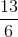Explanation:

In order to get rid of the radical, we square both sides: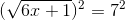Since the radical cancels out, we're left with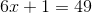Subtracting both sides by 1 gives us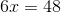We then divide both sides by 6 to get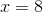Example Question #1 : Radical Functions

Which of the following is a solution to the following equation?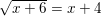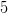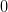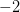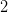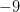Explanation:

We begin by sqaring both sides of the equation.  On the left side, the square root simply disappears, while on the right side we square the term.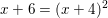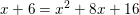We then set the left side equal to 0 by subtracting everything on that side.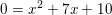We then factor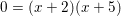Therefore,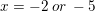With problems of this type, it is always wise to double check for any extraneous roots (answers that don't actually work for some reason).  However, in this case both answers work.  Sinceis the only option among our choices, we should go with it.

Example Question #1 : Solve Radical Equations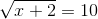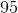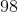Explanation:

When dealing with a radical equation, do the inverse operation to isolate the variable. In this case, the inverse operation of a square root is to square the expression. Thus we square both sides to continue. This yields the following.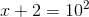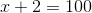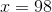Example Question #5 : Radical Functions

Solve: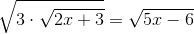Explanation:

1) To remove the radicals, raise both sides of the equation to the second power: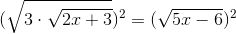2) To remove the radical, raise both side of the equation to the second power: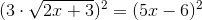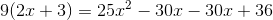3) Now simplify, write as a quadratic equation, and solve: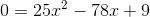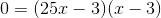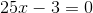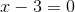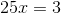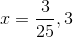4) Checking for extraneous solutions.

Plugging in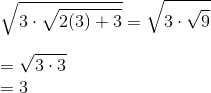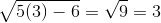Plugging in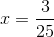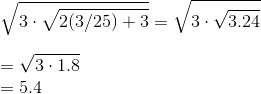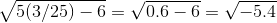Since the square root of negative 5.4 gives us an imaginary solution we conclude that the only real solution is x=3.

Example Question #1 : Radical Functions

Solve the rational equation: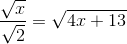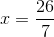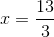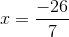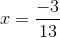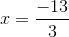Explanation:Square both sides to eliminate all radicals: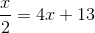Multiply both sides by 2: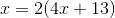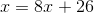Combine and isolate x: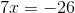Example Question #7 : Radical Functions

Solve this radical function: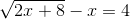Explanation: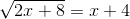Square both sides: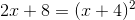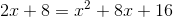Simplify: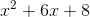Factor and set equal to zero: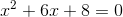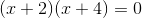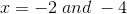All Precalculus Resources